## Deepika Singh

teacher | Maths Physics Chemistry Biology Zoology

### Description

I am a M.Sc, Physics postgraduate teacher teaches Physics, Chemistry, Maths, Science, etc ranging from Class VI to Class XII in all English and Hindi boards.

M.Sc

5-7 Years

11-12

### Current Organization

BHU

There are several examples, which reveal that the surface of a solid has the tendency to attract and retain the molecules of the phase with which it comes into contact. These molecules remain only at the surface and do
not go deeper into the bulk. The accumulation of molecular species at the surface rather than in the bulk of a solid or liquid is termed adsorption. The molecular species or substance, which concentrates or accumulates at the surface is termed adsorbate and the material on the surface of which the adsorption takes place is called adsorbent.
Adsorption is essentially a surface phenomenon. Solids, particularly in a finely divided state, have a large surface area and therefore, charcoal, silica gel, alumina gel, clay, colloids, metals in a finely divided state, etc.
act as good adsorbents.
(i) If a gas like O2, H2, CO, Cl2, NH3 or SO2 is taken in a closed vessel containing powdered charcoal, it is observed that the pressure of the gas in the enclosed vessel decreases. The gas molecules concentrate at the surface of the charcoal, i.e., gases are adsorbed at the surface.
(ii) In a solution of an organic dye, say methylene blue, when animal charcoal is added and the solution is well shaken, it is observed that the filtrate turns colourless. The molecules of the dye, thus,
accumulate on the surface of the charcoal, i.e., are adsorbed.

(iii) An aqueous solution of raw sugar, when passed over beds of animal charcoal, becomes colourless as the colouring substances are adsorbed by the charcoal.
(iv) The air becomes dry in the presence of silica gel because the water molecules get adsorbed on the surface of the gel. It is clear from the above examples that solid surfaces can hold the gas or liquid molecules by virtue of adsorption. The process of removing an adsorbed substance from a surface on which it is adsorbed is called desorption.

Solubility of a Solid in a Liquid

Every solid does not dissolve in a given liquid. While sodium chloride and sugar dissolve readily in water, naphthalene and anthracene do not. On the other hand, naphthalene and anthracene dissolve readily in benzene but sodium chloride and sugar do not. It is observed that polar solutes dissolve in polar solvents and nonpolar solutes in nonpolar solvents. In general, a solute dissolves in a solvent if the intermolecular interactions are similar in the two or we may say like dissolves like. When a solid solute is added to the solvent, some solute dissolves and its concentration increases in solution. This process is known as dissolution. Some solute particles in solution collide with the solid solute particles and get separated out of solution. This process is known as crystallization. A stage is reached when the two processes occur at the same rate. Under such conditions, a number of solute particles going into the solution will be equal to the solute particles separating out and a state of dynamic equilibrium is reached.

Solute + Solvent --> Solution

At this stage, the concentration of solute in solution will remain constant under the given conditions, i.e., temperature and pressure. A similar process is followed when gases are dissolved in liquid solvents. Such a solution in which no more solute can be dissolved at the same temperature and pressure is called a saturated solution. An unsaturated solution is one in which more solute can be dissolved at the same temperature. The solution which is in dynamic equilibrium with undissolved solute is the saturated solution and contains the maximum amount of solute dissolved in a given amount of solvent. Thus, the concentration of solute in such a solution is its solubility. Earlier we have observed that solubility of one substance into another depends on the nature of the substances. In addition to these
variables, two other parameters, i.e., temperature and pressure also control this phenomenon.

Effect of temperature The solubility of a solid in a liquid is significantly affected by temperature changes. Consider the equilibrium represented by the equation . This, being dynamic equilibrium, must follow Le Chateliers Principle. In general, if in a nearly saturated solution, the dissolution process is endothermic (Δsol H > 0), the solubility should increase with rising in temperature and if it is exothermic (Δsol H > 0) the solubility should decrease. These trends are also observed experimentally.

Effect of pressure Pressure does not have any significant effect on the solubility of solids in liquids. It is so because solids and liquids are highly incompressible and practically remain unaffected by changes in pressure

Capacitance - Spherical Conductor, Cylindrical Conductor

CAPACITANCE

Capacitance of a conductor is a measure of ability of the conductor to store charge on it. When a conductor is charged then its potential rises. The increase in potential is directly proportional to the charge given to the conductor.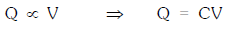The constant C is known as the capacity of the conductor.

Capacitance is a scalar quantity with dimension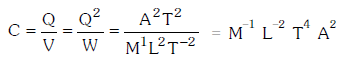Unit: - farad, coulomb/volt

The capacity of a conductor is independent of the charge given or its potential raised. It is also independent of nature of material and thickness of the conductor. Theoretically infinite amount of charge can be given to a conductor. But practically the electric field becomes so large that it causes ionisation of medium surrounding it. The charge on conductor leaks reducing its potential.

CAPACITANCE OF A SPHERICAL CONDUCTOR

When a charge Q is given to an isolated spherical conductor then its potential rises.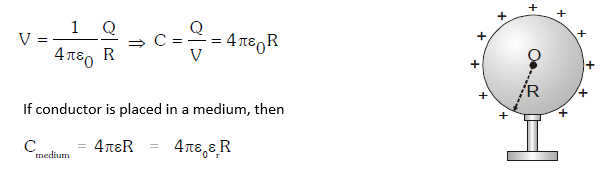Capacitance depends upon:

• Size and Shape of Conductor

• Surrounding medium

• Presence of other conductors nearby

CAPACITANCE OF A CYLINDRICAL CONDUCTOR

When a charge Q is given to inner cylinder it is uniformly distributed on its surface. A charge –Q is induced on inner surface of outer cylinder. The charge +Q induced on outer surface of outer cylinder flows to earth as it is grounded.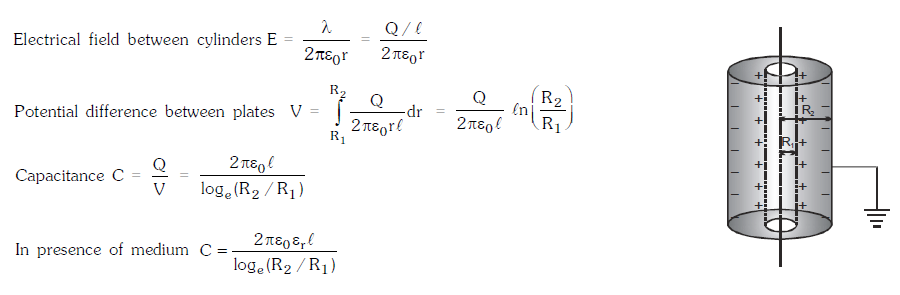CAPACITANCE OF A PAR ALLEL PL ATE CONDUCTOR

It consists of two metallic plates M and N each of area A at separation d. Plate M is positively charged and plate N is earthed. If r is the dielectric constant of the material medium and E is the field at a point P that exists between the two plates, then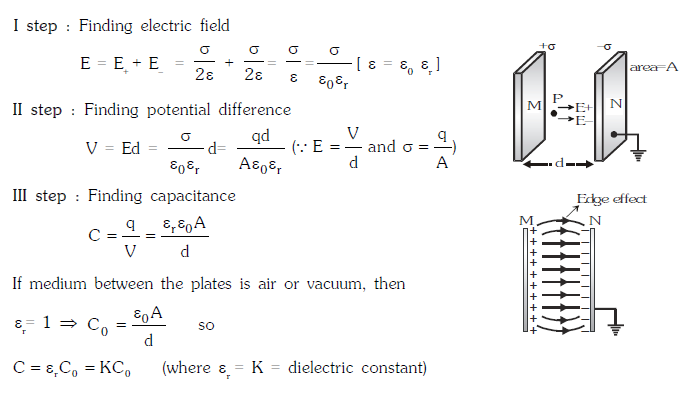Electric Charge – How a charge can be produced and Ways of charging a body

Electric Charge – How a charge can be produced and Ways of charging a body.

An electric charge is the property of substance so as to it feels force of attraction or repulsion due to interaction with another charge.

There are two types of electric charge

Positive charge

Negative charge

Electrons having negative charge whereas proton having positive charge. Same charges repel one another and opposite charges attract.

How a charge can be produced?

All bodies consist of atoms which contain equal amount of positive and negative charges in the form of protons and electrons respectively. The number of electrons being equal to the number of protons as an atom is neutral. If the electrons are removed from a body it gets +vely charged.

Ways of charging a body:

Charging by Friction:

When two bodies are rubbed together, a transfer of electrons take place from one body to another. The body from which electrons have been transferred is left with an excess of +ve charge so it gets positively charged and the body which receives the electrons becomes -vely charged. The +ve and –ve charges produced by rubbing are always equal in magnitude.

Charging by electrostatic induction:If a +vely charged rod is brought near an insulated conductor, the –ve charges (electrons) in the conductor will be attracted towards the rod. As a result, there will be an excess of –ve charge at the end of the conductor near the rod and the excessive +ve charge at the far end. This known as electrostatic induction.

The charges thus induced are found to be equal and opposite to each other. When the positively charged rod is removed, the conductor will again become electrically neutral.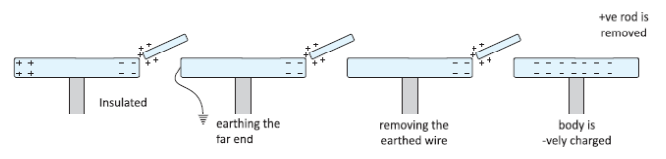Charging by conduction:

Take two conductors, one charged and other uncharged. Bring the conductors in contact with each other.

The charge (whether –ve or +ve) under its own repulsion will spread over both the conductors. Thus the conductors will be charged with the same sign. This is called as charging by conduction (through contact).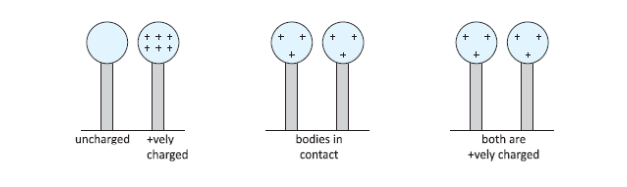Alternating Current, Average Current, R.M.S. Value of Alternating Current

ALTERNATING CURRENT

Alternating Current is a form of the electric power generated and mostly used in the world. The magnitude of alternating current changes continuously with time and its direction is reversed periodically. It is represented by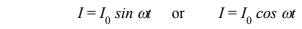where,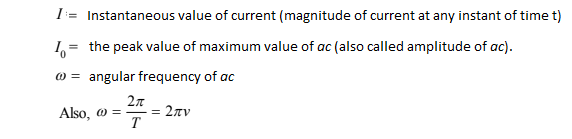where, T is the time period or period of ac It is equal to the time taken by the ac to go through one complete cycle of variation.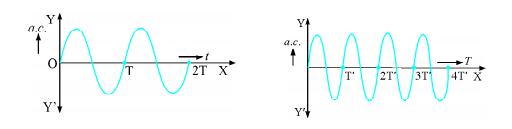The term used for ac holds equally for alternation voltage which may be represented by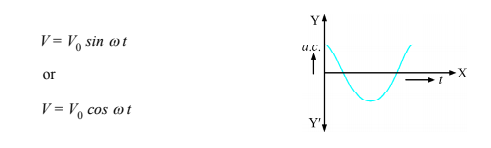In general,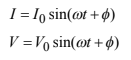Average Current (Mean Current)

As we know an alternating current is given byThe mean or average value of ac over any time t is given by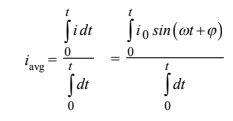In one complete cycle average current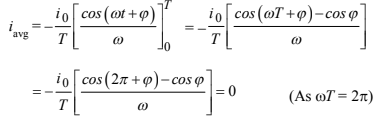Since ac is positive during the first half cycle and negative during the other half cycle so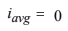Hence the dc instrument will indicate zero deflection when connected to a branch carrying ac current. So it is defined for either positive half cycle or negative half cycle.

Then, mean value of current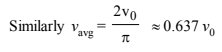R.M.S. Value of Alternating Current

The notation RMS refers to Root Mean Square, which is given by square root of mean of square current.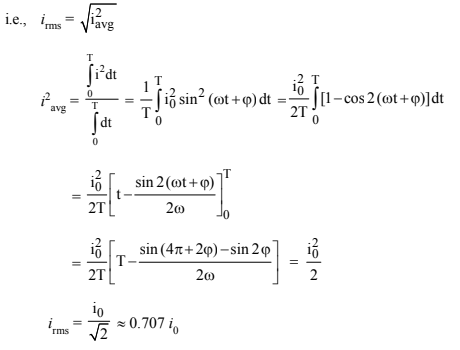Similarly, the RMS voltage is given by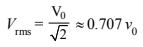The voltage across the resistor will be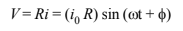The thermal energy developed in the resistor during the time t to t + dt is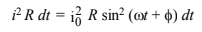The thermal energy developed in one-time period isSo in ac circuits, current and ac voltage are measured in terms of their rms values.

For eg. if house hold supply is 220V ac it means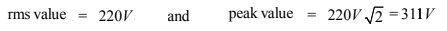No Active Challenge